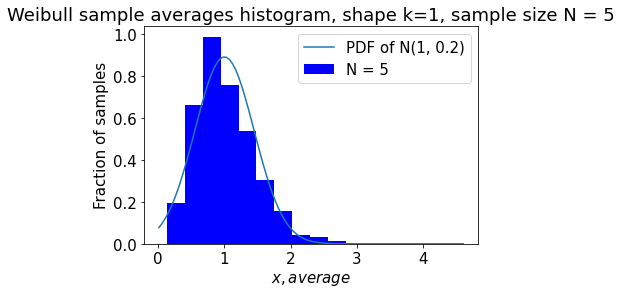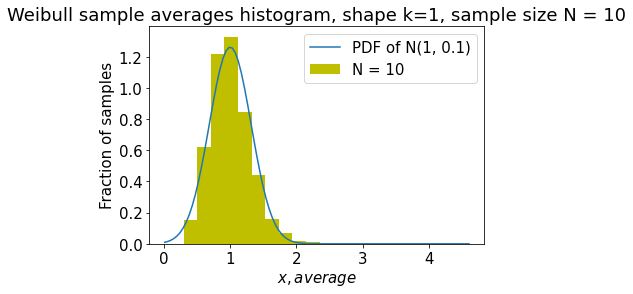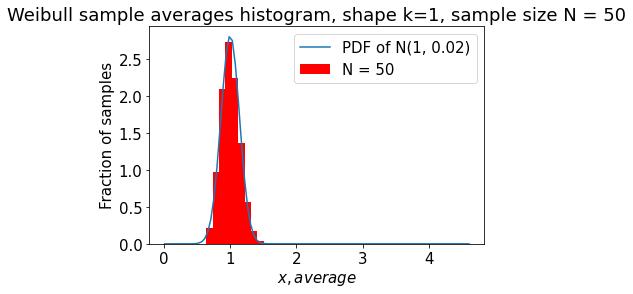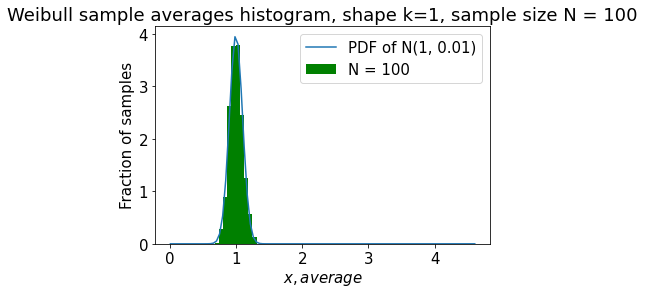Categories

## Weibull distribution & sample averages approximation using Python and scipy

In this post we share how to plot distribution histogram for the Weibull ditribution and the distribution of sample averages as approximated by the Normal (Gaussian) distribution. We’ll show how the approximation accuracy changes with samples volume increase.

One may get the full .ipynb file here.

Now we will estimate the distribution of the sample averages of a random Weibull variates for different sample sizes. To do this, we take fourN (5, 10, 50, 100), generate 1000 samples of volume N each and plot histograms of the distributions of their sample averages.

```N=[5, 10, 50, 100] # sample size
weibull_mean = {}
colors = iter(['b', 'y', 'r', 'g', 'pink']) # iterable colors
shape = 1
import math
for k in range(len(N)):
temp_mean = []
for i in range(1000):
weibull = np.random.weibull(shape, N[k])
temp_mean.append(np.mean(weibull))
weibull_mean[N[k]] = np.asarray(temp_mean)
# let's build histogram for Weibull sample averages
plt.hist(weibull_mean[N[k]], color=next(colors), density=True, label=f'N = {N[k]}')
plt.legend()
plt.ylabel('Fraction of samples')
plt.xlabel('\$x, average\$')
plt.title(f'Weibull sample averages histogram, shape k={shape}, sample size N = {N[k]}')

# let's build a corresponding Normal distribution PDF for the current N
norm_rv = sts.norm(loc = 1, scale = math.sqrt(1/N[k]))
pdf = norm_rv.pdf(x)
plt.plot(x, pdf, label=f'PDF of N(1, {1/N[k]})' )
plt.legend()
plt.show()
```This site uses Akismet to reduce spam. Learn how your comment data is processed.